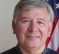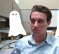## Data prediction in small embedded systemsStarted by 7 years ago10 replieslatest reply 7 years ago441 views

Hi.

I'm looking for methods to data forecast that can fit in small embedded systems (based in microcontrollers).

There are lots of methods based in different types of artificial neural network, but can be difficult to inplment this in small systems, principally to fit the training stage to the memory and processing power.

What methods can be better used in this context?

Some time ago I read saw something about predict data (time series) using digital filters, I don't remember if FIR or IIR filter, but I lost this reference. Does anyone know anything about it?

Other different methods will be very useful.

Thanks.

[ - ]If I might suggest, perhaps you'd be well advised to back away from the immediate objective focus: "fit in small embedded systems" and get to the functional objective.  Then, once you know what you could do to meet your objectives, you might tailor the computational approach to fit.  At this point it's just too vague to be able to usefully comment.

It's likely important to understand some approaches that are close to what you're trying to do.  One can make some rather arm-waving statements which could perhaps help you along the way:

Predicting data isn't likely to be done with a lowpass filter because it generally introduces a delay when, in fact, you're trying to generate a sequence that's *advanced* in time.  So, a highpass filter or, similarly, a differentiator is likely in the solution space.  Consider these:

- a predictor that uses the last 2 known values: x(n)=2*x(n-1) - x(n-2)
- a predictor that uses the last 3 known values: x(n)=3*x(n-1) - 3*x(n-2) + x(n-3)

The first one simply takes the slope between the last 2 values and extends it forward starting at the last known value.  It could be written
x(n)=x(n-1) + [x(n-1) - x(n-2)]
So, it's the known data plus the output of a rather classic +1,-1 differentiator.
The assumption or model is that the rate of change will hold.

The second one takes the same slope between the 2 past values and adds 3 intervals to the oldest of the two to project the prediction.  It could be written:
x(n)=x(n-3) + 3*[x(n-1)-x(n-2)]
So, it's still using a +1,-1 differentiator but projecting from a different point.
The assumption or model is that the outcome fits on a 2nd order curve.

Differentiation is a "noisy" process - just a well known observation that makes complete sense.  That is, if one differentiates a sequence, small differences are accentuated. Recognizing it as a highpass filter suggests the same conclusion.  Because of this, some practical differentiators will use as much lowpass filtering as practical before or after the differentiator.  It's a balance between too much noise in the output and fast enough response at the output.

Surely there will be noise in the data so this is something that you'd be trying to deal with both in the sense that it's filtered out and in the sense that it won't affect the prediction more than necessary.

As dudelsound points out, if you have a noisy set of data and no rules for their behavior then the best prediction is that the next value will be equal to the last value.  It's fairly easy to show that this is the case.  After all, the likely best data is the latest information and imposing some imagined model such as "the rate of change will hold" is conjecture that only adds errors.  The two models above would be doing this.

So, if you have a model that makes sense, you would likely want to use it.  But using a model when it has no basis except it "feels good" might not be good.  You have to examine your thinking carefully.

You can get more complicated and consider a Weiner-type filter (which is related to LMS algorithms, adaptive equalizers and noise cancellers) where somewhat stable spectral character can be important.  But my sense is that this is beyond what you might want to tackle.  It may also be too compute-intensive for your situation.  Nonetheless, an adapted noise canceller just a FIR filter.

[ - ]Fred Marshall, I agree with you. First I need to focus on the main question, or what method is possible to use, after this I can try to implement in the embedded system and optimize the code to fit in the restrictions.

There are lots of information in your answer, I need to "digest them".

Thanks

[ - ]This depends on what you're trying to predict. What is your data? For example if its a linear regression its not very computationally expensive...But you should be able to do some very complex math with some very afforable DSPs your only constraint is whether you'll have enough memory for your application and whether that memory can be accessed and processed fast enough to make your decision.

So what you need to know is what are you trying to predict and how fast do you need to predict it.

[ - ]Hi, sorry by the delay

Spetcavich, my data is related to energy consumption. For a "long" range, like one day or more, I think that a linear regression can be very interesting, but I will lose lots of information between this points. Using a microcontroller with dsp instructions I can do very complex math, the mainly limitation will be the memory.

The ideia is to fit the system in a Cortex M4 microcontroller, like the MSP432, Tiva or the STM32F429 (because of the additional external memory)

[ - ]So this energy consumption objective might be modeled based on temperature, predicted temperature, etc.  Depends on how the energy is being consumed of course.  So, anyway, that's a model.

The Kalman filter can be memory efficient so maybe that would be of interest.

[ - ]In order: Wiener filtering,  Kalmar filtering,  and neutral nets with the training done offline. Doing any kind of prediction without knowing the underlying process is probably unwise.
[ - ]Tim Wescott, the prediction is related to energy consumption.

I thought to test the kalman filter, but the Wiener filtering no, I will read about it.

I tried to predict some data using the ANN toolbox of the Matlab with the "Non linear autoregressive model (NAR)" and the result was very interesting, but works just using the toolbox, directly in the command line not (but this is due to some mistake of mine). Even working, I think that will be a little difficult to reproduce the same behaviour without know the internal functioning).

I read about other types os ANN (more simple), but I have not tested until this moment.

If using some ANN, the idea is to execute online training everytime that the error exceed some threshold.

[ - ]I would recommend that you first try to understand the physics (possibly in the form of circuit theory) that underlies the problem.  If you have a mathematical model of the physical system, a means of predicting what you want may just drop out of the math.

[ - ]As spetcavich said it all depends on what you want to predict. Prediction is only possible if you have some valid assumptions about the nature of the process you are trying to predict.

If you are trying to predict measurement signals that have a natural low-pass characteristic or tend to have periodic structures you could try to look up linear predictive coding (LPC) in wikipedia or ADPCM (adaptive differential pulse code modulation).

A very effective prediction of low-pass measurement data (like audio) in terms of performance versus cost would be:

X(n+1) = X(n)

So the prediction is "the value will not change". In an Audio file this can dramatically bring down your amplitudes if you are just storing the prediction error (real X(n+1) - predicted X(n+1)).

[ - ]